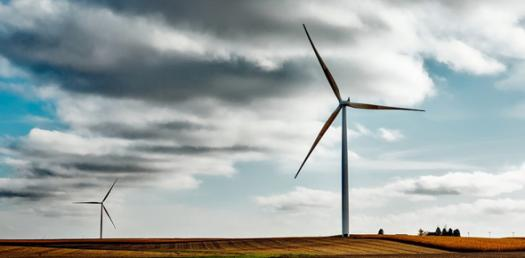# Wind Turbine Unit Exam

14 Questions | Total Attempts: 1409SettingsWind turbines are a global means of harnessing energy from the wind, as the kinetic energy is converted into electrical power for our use in machines, lights and so on. See how much you know about them with this quiz!

• 1.
Energy an object has due to it's motion is______________.
• A.

Potential energy

• B.

Kinetic energy

• C.

Gravitational energy

• 2.
Two balls are rolling at the same speed. One ball weighs more than the other. The heavier ball will have a __________ amount of kinetic energy.
• A.

Greater

• B.

Less

• C.

Equal

• 3.
A book is placed on a desk. As it falls off of the desk it is an example of ________________.
• A.

Kinetic energy converting into potential energy

• B.

Potential energy converting into kinetic energy

• 4.
A group of parts working together to achieve a desired goal is a _____________.
• A.

Cycle

• B.

Machine

• C.

System

• D.

Technology

• 5.
A system with feedback is a open loop system.
• A.

True

• B.

False

• 6.
What form of energy is the desired output of the wind turbine?
• A.

Electrical Energy

• B.

Mechanical Energy

• C.

Kinetic Energy

• D.

Solar Energy

• 7.
Wind turbines _______________ differnt forms of energy.
• A.

Create

• B.

Convert

• C.

Produce

• 8.
Which of the following will  not have an impact on the wind turbine designs output?
• A.

• B.

Rotor weight

• C.

• D.

Construction quality

• E.

None of the above, they will all have an effect the designs output

• 9.
This is an example of a ____________ axis wind turbine?
• A.

Vertical

• B.

Horizontal

• 10.
Which of the following could be positive impacts of wind energy?(Hint: you can select more than one)
• A.

It is GREEN energy

• B.

It creates jobs

• C.

It is loud

• D.

It reduces our dependency on petroleum (gas & oil)

• E.

It can disrupt bird migration

• F.

It can reduce energy costs

• 11.
___________ is the first step in the design process.
• A.

Gather information

• B.

Redesign & communicate

• C.

Understand the problem

• 12.
Why is the Redesign & Communicate step of the design process important?
• A.

It helps to gather information about the problem.

• B.

It allows designs to be continually improved.

• C.

It helps to test design solutions.

• 13.
The order of a system is _____________.
• A.

Output --> Input --> Process

• B.

Input --> Process --> Output

• C.

Input --> Output --> Process

• 14.
All technological systems have both positive and negative impacts.
• A.

True

• B.

False

Related TopicsBack to top